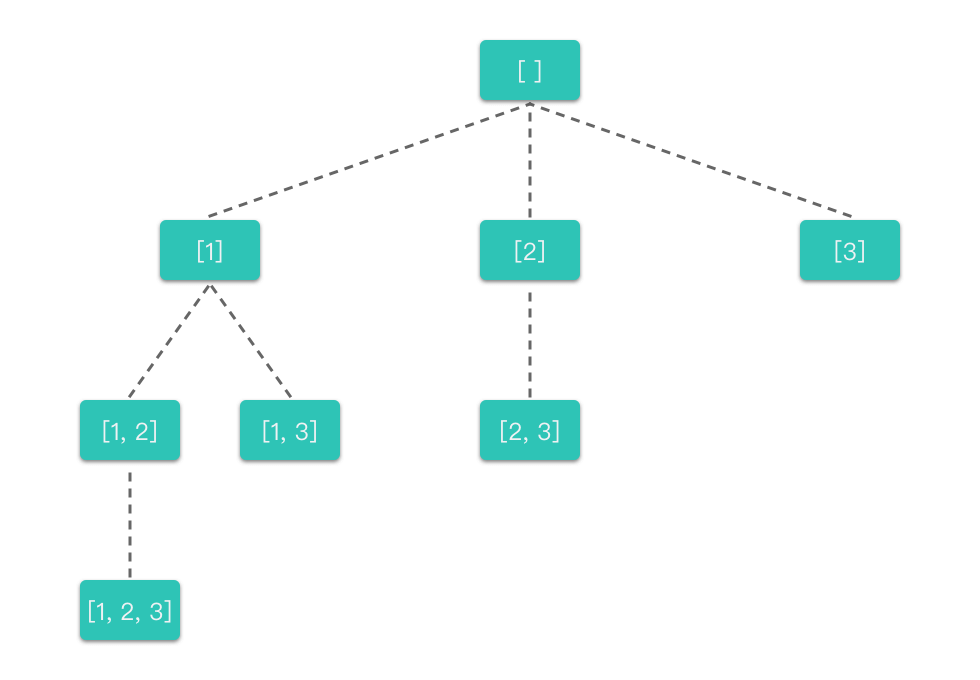0078. 子集

# 0078. 子集#

• 标签：位运算、数组、回溯
• 难度：中等

## 题目大意 #

• $1 \le nums.length \le 10$。
• $-10 \le nums[i] \le 10$。
• nums 中的所有元素互不相同。

• 示例 1：
 1 2  输入 nums = [1,2,3] 输出 [[],,,[1,2],,[1,3],[2,3],[1,2,3]] 
• 示例 2：
 1 2  输入：nums =  输出：[[],] 

## 解题思路 #

### 思路 1：回溯算法 #

1. 明确所有选择：根据数组中每个位置上的元素选与不选两种选择，画出决策树，如下图所示。
•1. 明确终止条件

• 当遍历到决策树的叶子节点时，就终止了。即当前路径搜索到末尾时，递归终止。
2. 将决策树和终止条件翻译成代码：

1. 定义回溯函数：

• backtracking(nums, index): 函数的传入参数是 nums（可选数组列表）和 index（代表当前正在考虑元素是 nums[i] ），全局变量是 res（存放所有符合条件结果的集合数组）和 path（存放当前符合条件的结果）。
• backtracking(nums, index): 函数代表的含义是：在选择 nums[index] 的情况下，递归选择剩下的元素。
2. 书写回溯函数主体（给出选择元素、递归搜索、撤销选择部分）。

• 从当前正在考虑元素，到数组结束为止，枚举出所有可选的元素。对于每一个可选元素：
• 约束条件：之前选过的元素不再重复选用。每次从 index 位置开始遍历而不是从 0 位置开始遍历就是为了避免重复。集合跟全排列不一样，子集中 {1, 2}{2, 1} 是等价的。为了避免重复，我们之前考虑过的元素，就不再重复考虑了。
• 选择元素：将其添加到当前子集数组 path 中。
• 递归搜索：在选择该元素的情况下，继续递归考虑下一个位置上的元素。
• 撤销选择：将该元素从当前子集数组 path 中移除。
 1 2 3 4  for i in range(index, len(nums)): # 枚举可选元素列表 path.append(nums[i]) # 选择元素 backtracking(nums, i + 1) # 递归搜索 path.pop() # 撤销选择 
1. 明确递归终止条件（给出递归终止条件，以及递归终止时的处理方法）。
• 当遍历到决策树的叶子节点时，就终止了。也就是当正在考虑的元素位置到达数组末尾（即 start >= len(nums)）时，递归停止。
• 从决策树中也可以看出，子集需要存储的答案集合应该包含决策树上所有的节点，应该需要保存递归搜索的所有状态。所以无论是否达到终止条件，我们都应该将当前符合条件的结果放入到集合中。

### 思路 1：代码 #

  1 2 3 4 5 6 7 8 9 10 11 12 13 14 15 16  class Solution: def subsets(self, nums: List[int]) -> List[List[int]]: res = [] # 存放所有符合条件结果的集合 path = [] # 存放当前符合条件的结果 def backtracking(nums, index): # 正在考虑可选元素列表中第 index 个元素 res.append(path[:]) # 将当前符合条件的结果放入集合中 if index >= len(nums): # 遇到终止条件（本题） return for i in range(index, len(nums)): # 枚举可选元素列表 path.append(nums[i]) # 选择元素 backtracking(nums, i + 1) # 递归搜索 path.pop() # 撤销选择 backtracking(nums, 0) return res 

### 思路 1：复杂度分析 #

• 时间复杂度：$O(n \times 2^n)$，其中 $n$ 指的是数组 nums 的元素个数，$2^n$ 指的是所有状态数。每种状态需要 $O(n)$ 的时间来构造子集。
• 空间复杂度：$O(n)$，每种状态下构造子集需要使用 $O(n)$ 的空间。

### 思路 2：二进制枚举 #

• 对于长度为 5 的集合 nums 来说，只需要枚举 $0 \sim 2^n - 1$（共 $2^n$ 种情况），即可得到所有的子集。

### 思路 2：代码 #

  1 2 3 4 5 6 7 8 9 10 11  class Solution: def subsets(self, nums: List[int]) -> List[List[int]]: n = len(nums) # n 为集合 nums 的元素个数 sub_sets = [] # sub_sets 用于保存所有子集 for i in range(1 << n): # 枚举 0 ~ 2^n - 1 sub_set = [] # sub_set 用于保存当前子集 for j in range(n): # 枚举第 i 位元素 if i >> j & 1: # 如果第 i 为元素对应二进制位为 1，则表示选取该元素 sub_set.append(nums[j]) # 将选取的元素加入到子集 sub_set 中 sub_sets.append(sub_set) # 将子集 sub_set 加入到所有子集数组 sub_sets 中 return sub_sets # 返回所有子集 

### 思路 2：复杂度分析 #

• 时间复杂度：$O(n \times 2^n)$，其中 $n$ 指的是数组 nums 的元素个数，$2^n$ 指的是所有状态数。每种状态需要 $O(n)$ 的时间来构造子集。
• 空间复杂度：$O(n)$，每种状态下构造子集需要使用 $O(n)$ 的空间。